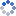加载中…氯化钡和硫酸银
• 博客等级：• 博客积分：0
• 博客访问：91,221
• 关注人气：37
• 获赠金笔：0支
• 赠出金笔：0支
• 荣誉徽章：

## 连分数简介（4）：无限简单连分数(2014-06-23 19:42:45)
 分类： 数学趣题-代数-数论

有理数用有限简单连分数表示，如果试着对无理数去辗转相除，会发现除不尽，这启示我们是不是可以把有限连分数推广到无限连分数来表示无理数。我们引进无限简单连分数的概念：

设 a_0,a_1,a_2,a_3,... 为一个无穷数列，第一项 a_0 是整数，其余项是正整数，则极限的值称为无限简单连分数，记作当然，如果只限定“除第一项之外所有项都是正数”的条件，这个值就叫做无限连分数。无限连分数不一定有意义（极限不一定存在），此时称该无限连分数发散，否则称该无限连分数收敛。

同理，我们把 [a_0,a_1,a_2,a_3,...,a_n] 叫做该无限连分数的第 n 个渐近分数

我们先证明无限简单连分数一定收敛并且是无理数，为此记无限简单连分数 [a_0,a_1,a_2,a_3,...] 的第 n 个渐近分数为 s_n ，我们需要证明数列 {s_n} 的极限存在并且是无理数。

这里我们可以判断各个 s_n 的大小关系：于是数列 s_0,s_2,s_4,s_6,... 单调递增，并且有上界 s_1 ，故该数列必然有极限，极限值记为 r' 。同理 s_1,s_3,s_5,s_7,... 单调递减，并且有下界 s_0 ，该数列也有极限，极限值记为 r'' 。

又由于 s_n-s_(n-1)=(-1)^(n+1)/(q_n*q_(n-1)) ，我们得到但是数列 {q_n}(n=1,2,3,...) 是严格单调递增的，所以它的极限必为正无穷大，也就是说左边可以拆成 r''-r' ，故 r' 和 r'' 是相等的。这个值就是数列 {s_n} 的极限。设该极限为 r_0 ，则显然有假设 r_0 为有理数，则它可以写成 p/q 的形式，其中 p 为整数，q 为正整数，(p,q)=1 。对任意非负整数 n 有下式成立：也就是说由于 uq_n-vp_n 一定是整数，故 |uq_n-vp_n| 必然大于等于 1 ，也就是说也就是说 q_(n+1) 小于 v ，但是数列 {q_n}(n=1,2,3,...) 的极限是正无穷大，所以 q_(n+1) 不可能永远小于 v ，矛盾。因此 r_0 为无理数。

接下来研究无理数化为无限简单连分数的问题，为此先引入一个记号：记为无限连分数 [a_0,a_1,a_2,a_3,...] 的第 n 个完全商。显然第 0 个完全商就是它自己。

其中 n 为非负整数。回想我们把有理数化为有限简单连分数，用的是不断“取出整数部分再取倒数”的方法。这里由于是无限简单连分数，就没有最后一个数是不是 1 的问题了，我们可以证明对 n=0,1,2,3,...这是由于，根据无限连分数定义有位于分母上的连分数由于各项全为正整数，所以必然大于 0 ，也就是说同时我们还可以得到 r_n(n=1,2,3,...) 均大于 1 （因为 r_n 大于正整数 a_n ）。于是对任意非负整数 n 均有 1/r_(n+1) 大于 0 小于 1 ，而 a_n 又是整数，故必有 a_n=[r_n] 。

这个式子也可以写成也就是说我们可以递推得这样两个式子就都证完了。我们还可以直接推出无理数最多能表示为一个无限简单连分数。这是由于我们已经证明了：因此给定无理数 r_0 则数列 {a_n},{r_n} 均被唯一确定，所以无理数 r_0 的无限简单连分数表示最多只有这一种。只要证明这样求出的数列 {a_n} 对应的无限简单连分数恰好等于 r_0 ，我们就证明出了任意一个无理数都可以唯一表示为一个无限简单连分数

我们把这个结论重新叙述一遍：设 r_0 为任意无理数，定义数列 {a_n},{r_n}(n=0,1,2,3,...) 满足那么 [a_0,a_1,a_2,a_3,...] 是无限简单连分数且值为 r_0 。

首先证明 [a_0,a_1,a_2,a_3,...] 是无限简单连分数，由于已知 a_n=[r_n] 是整数，只需证明对任意正整数 n 都有 a_n 为正整数，这是因为 r_n-a_n 是 r_n 的小数部分，一定大于 0 小于 1 （它不可能等于 0 否则逆推回去可得 r_0 为有理数，矛盾），所以 r_(n+1) 大于 1 ，所以 a_n 是正整数。

然后由于 r_n=a_n+1/r_(n+1)=[a_n,r_(n+1)] ，我们就可以递推得到由我们关于有限连分数比较大小的结论可知另外我们已经会比较各个渐近分数 s_n ，我们知道记 {s_n} 偶数项极限为 r' ，奇数项极限为 r'' ，显然 r_0 不会小于 r' （否则 r_0 会小于 {s_n} 的某个偶数项，这是不可能的），也不会大于 r'' （否则 r_0 会大于 {s_n} 的某个奇数项）。但是，我们已经证过了 r',r'' 是一回事，都等于无限简单连分数 [a_0,a_1,a_2,a_3,...] 的值。所以只可能 r_0=r'=r'' ，也就是说 r_0 就是无限简单连分数 [a_0,a_1,a_2,a_3,...] 的值。这就证完了。

对于一个给定的无理数 r_0 ，我们理论上可以通过递推（a_n=[r_n],r_(n+1)=1/(r_n-a_n) ）求出 r_0 无限简单连分数表示的任意多位。

接下来说点正事，我们说连分数的一个重要用途就是求近似分数，我们这就讨论无限简单连分数的渐近分数作为有理数逼近的误差（这里误差指渐近分数与原无理数 r_0 的差的绝对值，为了不打那么多字...）。

先给误差估个范围：设 r_0 为无理数，s_n=p_n/q_n 为第 n 个渐近分数，则有证明的关键是利用式子这个式子用过好几次了，如果还看不出的话把 r_(n+1) 看成 a_(n+1) 就看出来了吧...

于是就可以算出由于 r_(n+1) 这个变量是多余的，所以我们通过放缩把它去掉。注意到代到上式里面很容易得到嗯，这个还没算两步就出来了...由这个可以得到一些推论，比如如果考虑相邻两渐近分数的误差就得到：说明越靠后的渐近分数误差越小。

我们说过连分数的渐近分数是比较优的渐近分数，现在我们就叙述并证明这一点：若 n 为正整数，则 s_n=p_n/q_n 是一切分母不超过 q_n 且为正整数的分数中与 r_0 最接近的。（为啥限定 n 不为 0 ，举个例子，例如 e=[2,1,2,...] 第 0 个渐近分数是 2/1 ，但是显然 3/1 更接近 e ）也就是说，对于 n>=1 任意整数 a,b （ b 为小于 q_n 的正整数）都有不过，事实上这个式子还是有些弱，我们把它改成这样：由第二个式子可以推出第一个式子（注意 1/b 大于等于 1/q_n ，同向正不等式可相乘），反过来则推不出来，我们只需证明第二个式子就行了。

利用反证法，我们只需证明若则 b 一定大于 q_n 。我们再次加强命题，把“大于 q_n ”改成“大于等于 q_(n+1) ”。当 n 为正整数时有 q_(n+1) 大于 q_n ，因此这确实是加强命题（至少没有减弱）。

事实上，加强后的命题当 n=0 时也成立（有完没完了...），因此我们只需证明这个结论：

若整数 a 和正整数 b 满足则 b 一定大于等于 q_(n+1) 。

证明的关键是让 a,b 与渐近分数联系起来，考虑关于 x,y 的方程组把它解出来是这样的：不过，别忘了分母一定等于 1 或 -1 ，所以事实上这是一组整数解：这个式子暂时没啥用，反正我们知道 x,y 是整数，接下来我们把已知不等式中的 a,b 全部换掉：也就是说已知条件变成了这样：左右一对照发现有相同的部分，假设左边加号两边的两个式子都不为 0 且同号，我们就可以这样计算：这个式子不超过 |q_n*r_0-p_n| （这里利用了 x 为整数的条件，这样能保证 |x| 不小于 1 ），这和已知不等式矛盾。

注意到 q_n*r_0-p_n 与 q_(n+1)*r_0-p_(n+1) 一定不等于 0 且异号（这是由于 r_0 的相邻两个渐近分数必然一个比 r_0 大，另一个比 r_0 小），所以上面的假设就等价于 x,y 异号。于是这个矛盾说明了 x,y 不可能异号。

再注意到，若 x 不为零且 y 等于零，上面的推理仍然成立，同样产生矛盾。所以这种情况也不可能。所以我们就得到了：要么 x 等于零，要么 x,y 同号。

如果 x=0 ，代到一开始的那个方程组里，得到 b=q_(n+1)*y ，所以正整数 b 为 q_(n+1) 的整数倍（这里利用了 y 为整数），显然大于等于 q_(n+1) 。

如果 x,y 同号，我们仍然使用式子 b=q_n*x+q_(n+1)*y ，显然 x,y 必然同为正数，同时我们立即得到 b 大于 q_(n+1) 。

不管怎样都有 q 大于等于 q_(n+1) ，这就证明了这个结论。

连分数产生的近似分数都是很好的逼近，那么反过来给一个足够好的逼近，能否说明它就是其中一个近似分数？我们有下面的结论：

若整数 a 和正整数 b 满足则 a/b 一定是 r_0 的某个渐近分数。

我们先给 b 卡个范围，考虑 r_0 的各个近似分数的分母组成的数列 {q_k}(k=0,1,2,3,...) ，该数列单调不减（即后一项不小于前一项）并且第一项一定为 1 ，这也就是说，对于任意正整数 b 一定存在唯一的非负整数 n 使得下式成立：由不等式的右半边，逆用刚才的定理，我们就得到了我们反设 b 不等于 q_n ，也就是说 a/b 不等于 p_n/q_n （当然前提是先把 a,b 的公约数越掉），我们可以得到下式：结合这几个式子就可以得到这样我们导出了矛盾。于是我们断定 b 一定等于 q_n 。接下来继续使用上面的连不等式，当然由于没有 b 不等于 q_n 的反设，上式的第一个不等号不成立，但是后面都是成立的，也就是说这也就是说这说明 a 恰好等于 p_n ，故 a/b=p_n/q_n 为 r_0 的一个渐近分数，结论证完。

这个结论将在利用连分数寻找 Pell 方程最小解时用到。

0